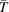International
Tables for
Crystallography
Volume C
Mathematical, physical and chemical tables
Edited by E. Prince

International Tables for Crystallography (2006). Vol. C, ch. 6.3, pp. 599-608
https://doi.org/10.1107/97809553602060000602

## Chapter 6.3. X-ray absorption

E. N. Maslena

aCrystallography Centre, The University of Western Australia, Nedlands, Western Australia 6009, Australia

### References

Abramowitz, M. & Stegun, I. A. (1964). Handbook of mathematical functions, p. 916. National Bureau of Standards Publication AMS 55.
Alcock, N. W., Pawley, G. S., Rourke, C. P. & Levine, M. R. (1972). An improvement in the algorithm for absorption correction by the analytical method. Acta Cryst. A28, 440–444.
Anderson, D. W. (1984). Absorption of ionizing radiation. Baltimore: University Park Press.
Azaroff, L. V., Kaplow, R., Kato, N., Weiss, R. J., Wilson, A. J. C. & Young, R. A. (1974). X-ray diffraction, pp. 282–284. New York: McGraw-Hill.
Becker, P. J. & Coppens, P. (1974). Extinction within the limit of validity of the Darwin transfer equations. I. General formalisms for primary and secondary extinction and their application to spherical crystals. Acta Cryst. A30, 129–147.
Beeman, W. W. & Friedman, H. (1939). The X-ray K absorption edges of the elements Fe (26) to Ge (32). Phys. Rev. 56, 392–405.
Coppens, P. (1970). The evaluation of absorption and extinction in single crystal structure analysis. Crystallographic computing, edited by F. R. Ahmed, S. R. Hall & C. P. Huber, pp. 255–270. Copenhagen: Munksgaard.
Coyle, B. A. (1972). Absorption and volume corrections for a cylindrical specimen, larger than the beam, and in general orientation. Acta Cryst. A28, 231–233.
Coyle, B. A. & Schroeder, L. W. (1971). Absorption and volume corrections for a cylindrical sample, larger than the X-ray beam, employed in Eulerian geometry. Acta Cryst. A27, 291–295.
Dwiggins, C. W. Jr (1975a). Rapid calculation of X-ray absorption correction factors for cylinders to an accuracy of 0.1%. Acta Cryst. A31, 146–148.
Dwiggins, C. W. Jr (1975b). Rapid calculation of X-ray absorption correction factors for spheres to an accuracy of 0.05%. Acta Cryst. A31, 395–396.
Flack, H. D. (1974). Automatic absorption correction using intensity measurements from azimuthal scans. Acta Cryst. A30, 569–573.
Flack, H. D. (1977). An empirical absorption–extinction correction technique. Acta Cryst. A33, 890–898
Flack, H. D. & Vincent, M. G. (1978). Absorption weighted mean path lengths for spheres. Acta Cryst. A34, 489–491.
Fukamachi, T., Karamura, T., Hayakawa, K., Nakano, Y. & Koh, F. (1982). Observation of effect of temperature on X-ray diffraction intensities across the In K absorption edge of InSb. Acta Cryst. A38, 810–813.
Graaff, R. A. G. de (1973). A Monte Carlo method for the calculation of transmission factors. Acta Cryst. A29, 298–301.
Graaff, R. A. G. de (1977). On the calculation of transmission factors. Acta Cryst. A33, 859.
James, R. W. (1962). The optical principles of the diffraction of X-rays, pp. 135–192. Ithaca: Cornell University Press.
Karamura, T. & Fukamachi, T. (1979). Temperature dependence of X-ray reflection intensity from an absorbing perfect crystal near an absorption edge. Acta Cryst. A35, 831–835.
Katayama, C., Sakabe, N. & Sakabe, K. (1972). A statistical evaluation of absorption. Acta Cryst. A28, 293–295.
Kopfmann, G. & Huber, R. (1968). A method of absorption correction for X-ray intensity measurements. Acta Cryst. A24, 348–351.
Krause, M. O. & Oliver, J. H. (1979). Natural widths of atomic K and L levels, Kα X-ray lines and several KLL Auger lines. J. Phys. Chem. Ref. Data, 8, 329–338.
Lee, B. & Ruble, J. R. (1977a). A semi-empirical absorption-correction technique for symmetric crystals in single-crystal X-ray crystallography. I. Acta Cryst. A33, 629–637.
Lee, B. & Ruble, J. R. (1977b). A semi-empirical absorption-correction technique for symmetric crystals in single-crystal X-ray crystallography. II. Acta Cryst. A33, 637–641.
North, A. C. T., Phillips, D. C. & Mathews, F. S. (1968). A semi-empirical method of absorption correction. Acta Cryst. A24, 351–359.
Rigoult, J. & Guidi-Morosini, C. (1980). An accurate calculation ofμ for spherical crystals. Acta Cryst. A36, 149–151.
Rouse, K. D., Cooper, M. J., York, E. J. & Chakera, A. (1970). Absorption corrections for neutron diffraction. Acta Cryst. A26, 682–691.
Santoro, A. & Wlodawer, A. (1980). Absorption corrections for Weissenberg diffractometers. Acta Cryst. A36, 442–450.
Schwager, P., Bartels, K. & Huber, R. (1973). A simple empirical absorption-correction method for X-ray intensity data films. Acta Cryst. A29, 291–295.
Stroud, A. H. & Secrest, D. (1966). Gaussian quadrature formulas. New Jersey: Prentice-Hall.
Stuart, D. & Walker, N. (1979). An empirical method for correcting rotation-camera data for absorption and decay effects. Acta Cryst. A35, 925–933.
Templeton, D. H. & Templeton, L. K. (1980). Polarized X-ray absorption and double refraction in vanadyl bisacetylacetonate. Acta Cryst. A36, 237–241.
Templeton, D. H. & Templeton, L. K. (1982). X-ray dichroism and polarized anomalous scattering of the uranyl ion. Acta Cryst. A38, 62–67.
Templeton, D. H. & Templeton, L. K. (1985). Tensor optical properties of the bromate ion. Acta Cryst. A41, 133–142.
Tibballs, J. E. (1982). The rapid computation of mean path lengths for cylinders and spheres. Acta Cryst. A38, 161–163.
Wagenfeld, H. (1975). Theoretical computations of X-ray dispersion corrections. Anomalous scattering, edited by S. Ramaseshan & S. C. Abrahams, pp. 13–24. Copenhagen: Munksgaard.
Walker, N. & Stuart, D. (1983). An empirical method for correcting diffractometer data for absorption effects. Acta Cryst. A39, 158–166.
Weber, K. (1969). Eine neue Absorptionsfactortafel für kugelförmige Proben. Acta Cryst. B25, 1174–1178.
Zachariasen, W. H. (1968). Extinction and Borrmann effect in mosaic crystals. Acta Cryst. A24, 421–424.